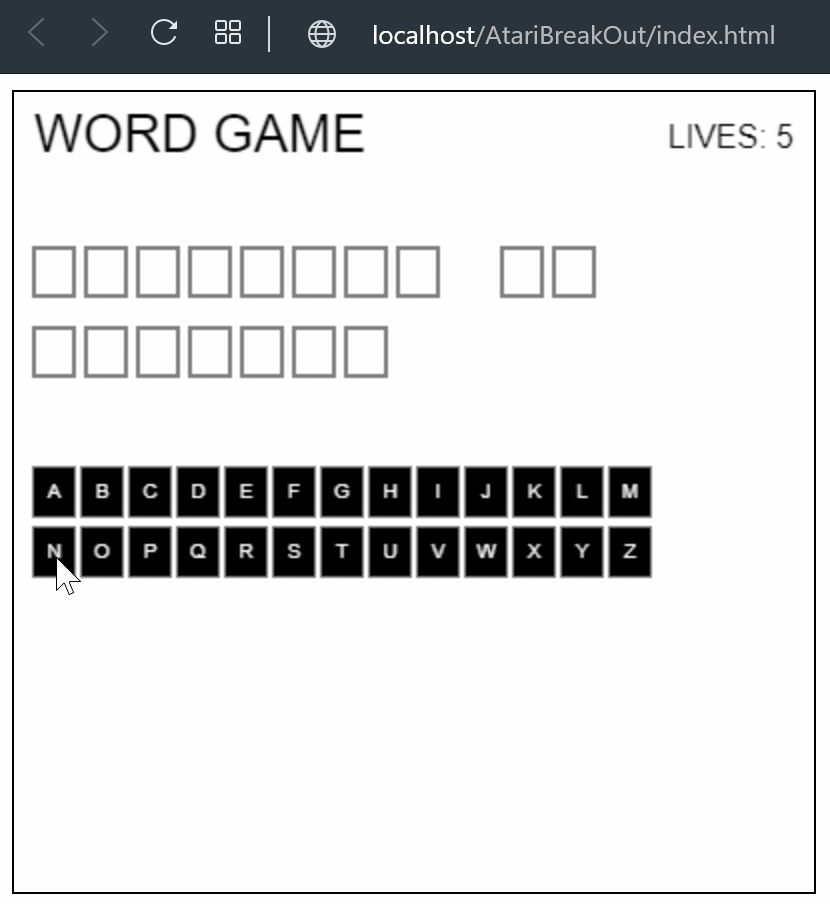# Games[BANanoCreateJS] Word Game

#### Mashiane

##### Expert
Ola

BANanoCreateJS

The word game is a simple HTML5 based game developed with a BANano based library named BANanoCreateJS wrapping the CreateJS library.

Here one is given 5 lives and words to guess. Each time a wrong character is chosen the number of lives decreased.When a right character is selected, its removed from available letters and added to the correct letters. The game is running at 60 FPS.

Ta.

PS: The right answer is CreateJS Is Awesome!, which will show, YOU WIN!

Source code for the game is on the link above!

•ilan

#### Mashiane

##### Expert
The game is developed by drawing the shapes, then letters for each alphabet and assigning a click event to the boxes.

B4X:
``````Sub game_ready
drawBoard
drawLetters
drawMessages
startGame
End Sub``````

B4X:
``````'these are all the words we need
Sub drawBoard
Dim schar As String
Dim i As Int
Dim xPos As Int = 20
Dim yPos As Int = 90
Dim abcLen As Int = answer.Length - 1
'
For i = 0 To abcLen
If (schar <> " ") And (schar <> "&") Then
lettersNeeded = lettersNeeded + 1
Dim box As CreateJSShape
box.Initialize
box.graphics.beginStroke("#000")
box.graphics.drawRect(0, 0, 20, 24)
box.SetRegX(10)
box.SetRegY(12)
box.SetX(xPos)
box.SetY(yPos)
box.SetName("box_" & i)
box.SetKey(schar)
End If
xPos = xPos + 26
If schar = "&" Then
yPos = yPos + 40
xPos = 20
End If
Next
End Sub``````

Boxes for the correct sentence to guess are created.

Then draw the letters from A-Z.

B4X:
``````Sub drawLetters
Dim i As Int
Dim schar As String
Dim cnt As Int = 0
Dim xPos As Int = 20
Dim yPos As Int = 200
Dim abcLen As Int = abc.length - 1
For i = 0 To abcLen
schar = abc.SubString2(i,i+1)
Dim btn As CreateJSShape
btn.initialize
btn.graphics.beginFill("#000")
btn.graphics.beginStroke("#000")
btn.graphics.drawRect(0, 0, 20, 24)
btn.regX(10)
btn.regY(12)
btn.setx(xPos)
btn.sety(yPos)

'create text
Dim txt As CreateJSText
txt.initialize1(schar)
txt.setcolor("#FFF")
txt.settextAlign("center")
txt.settextBaseline("middle")
txt.setx(xPos)
txt.sety(yPos)
'
btn.Settxt(txt.Text)
Dim e As BANanoEvent
xPos = xPos + 24
cnt = cnt + 1
If (cnt = 13) Then
yPos = yPos + 30
xPos = 20
End If
Next
End Sub``````

When a letter is clicked, we check for a match on the sentence to guess and also update the game life score.

B4X:
``````Sub onLetterClick(e As BANanoEvent)
Dim btn As BANanoObject = game.GetEventTarget(e)
Dim txt As BANanoObject = btn.GetField("txt")
'Dim e As BANanoEvent
'btn.removeEventListener("click", BANano.CallBack(Me, "onLetterClick", Array(e)))
checkForMatches(txt)
checkGame
End Sub``````

Based on a win / loose, the results are tweened

B4X:
``````Sub gameOver() {
game.removeAllChildren
Dim msg As String
If win Then
msg = "YOU WIN!"
Else
msg = "YOU LOSE"
End If
gameOverTxt.Initialize2(msg, "36px Arial")
gameOverTxt.Setalpha(0)
Dim scolor As String
If win Then
scolor = "true"
Else
scolor = "red"
End If
gameOverTxt.Setcolor(scolor)
gameOverTxt.SettextAlign("center")
gameOverTxt.SettextBaseline("middle")
gameOverTxt.Setx(game.GetCanvasWidth / 2)
gameOverTxt.Sety(game.Getcanvasheight / 2)
game.Tween_Get(gameOverTxt.text,False)
game.Tween_To2(CreateMap("alpha":1),1000)
End Sub``````

•Ronce and ilan